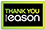local_shipping Spend over €10 for free home delivery  place Free 2 Hour Click & Collect Service

# Stochastic partial differential equations

Category: Mathematics
Explore Theory and Techniques to Solve Physical, Biological, and Financial Problems Since the first edition was published, there has been a surge of interest in stochastic partial differential equations (PDEs) driven by the Lévy type of noise. Stochastic Partial Differential Equations, Second Edition incorporates these recent developments and improves the presentation of material. New to the Second Edition Two sections on the Lévy type of stochastic integrals and the related stochastic differential equations in finite dimensions Discussions of Poisson random fields and related stochastic integrals, the solution of a stochastic heat equation with Poisson noise, and mild solutions to linear and nonlinear parabolic equations with Poisson noises Two sections on linear and semilinear wave equations driven by the Poisson type of noises Treatment of the Poisson stochastic integral in a Hilbert space and mild solutions of stochastic evolutions with Poisson noises Revised proofs and new theorems, such as explosive solutions of stochastic reaction diffusion equations Additional applications of stochastic PDEs to population biology and finance Updated section on parabolic equations and related elliptic problems in Gauss-Sobolev spaces The book covers basic theory as well as computational and analytical techniques to solve physical, biological, and financial problems. It first presents classical concrete problems before proceeding to a unified theory of stochastic evolution equations and describing applications, such as turbulence in fluid dynamics, a spatial population growth model in a random environment, and a stochastic model in bond market theory. The author also explores the connection of stochastic PDEs to infinite-dimensional stochastic analysis.
€106.39319 Reward Points
Currently out of stock
Delivery 5-7 Days

Any purchases for more than €10 are eligible for free delivery anywhere in the UK or Ireland!

Explore Theory and Techniques to Solve Physical, Biological, and Financial Problems Since the first edition was published, there has been a surge of interest in stochastic partial differential equations (PDEs) driven by the Lévy type of noise. Stochastic Partial Differential Equations, Second Edition incorporates these recent developments and improves the presentation of material. New to the Second Edition Two sections on the Lévy type of stochastic integrals and the related stochastic differential equations in finite dimensions Discussions of Poisson random fields and related stochastic integrals, the solution of a stochastic heat equation with Poisson noise, and mild solutions to linear and nonlinear parabolic equations with Poisson noises Two sections on linear and semilinear wave equations driven by the Poisson type of noises Treatment of the Poisson stochastic integral in a Hilbert space and mild solutions of stochastic evolutions with Poisson noises Revised proofs and new theorems, such as explosive solutions of stochastic reaction diffusion equations Additional applications of stochastic PDEs to population biology and finance Updated section on parabolic equations and related elliptic problems in Gauss-Sobolev spaces The book covers basic theory as well as computational and analytical techniques to solve physical, biological, and financial problems. It first presents classical concrete problems before proceeding to a unified theory of stochastic evolution equations and describing applications, such as turbulence in fluid dynamics, a spatial population growth model in a random environment, and a stochastic model in bond market theory. The author also explores the connection of stochastic PDEs to infinite-dimensional stochastic analysis.
Currently out of stock
Delivery 5-7 Days319 Reward Points

Any purchases for more than €10 are eligible for free delivery anywhere in the UK or Ireland!

€106.39
Currently out of stock
Delivery 5-7 Days319 Reward Points

Any purchases for more than €10 are eligible for free delivery anywhere in the UK or Ireland!

### Product Description

Explore Theory and Techniques to Solve Physical, Biological, and Financial Problems Since the first edition was published, there has been a surge of interest in stochastic partial differential equations (PDEs) driven by the Lévy type of noise. Stochastic Partial Differential Equations, Second Edition incorporates these recent developments and improves the presentation of material. New to the Second Edition Two sections on the Lévy type of stochastic integrals and the related stochastic differential equations in finite dimensions Discussions of Poisson random fields and related stochastic integrals, the solution of a stochastic heat equation with Poisson noise, and mild solutions to linear and nonlinear parabolic equations with Poisson noises Two sections on linear and semilinear wave equations driven by the Poisson type of noises Treatment of the Poisson stochastic integral in a Hilbert space and mild solutions of stochastic evolutions with Poisson noises Revised proofs and new theorems, such as explosive solutions of stochastic reaction diffusion equations Additional applications of stochastic PDEs to population biology and finance Updated section on parabolic equations and related elliptic problems in Gauss-Sobolev spaces The book covers basic theory as well as computational and analytical techniques to solve physical, biological, and financial problems. It first presents classical concrete problems before proceeding to a unified theory of stochastic evolution equations and describing applications, such as turbulence in fluid dynamics, a spatial population growth model in a random environment, and a stochastic model in bond market theory. The author also explores the connection of stochastic PDEs to infinite-dimensional stochastic analysis.

### Product Details

#### Stochastic partial differential equations

ISBN9781466579552

Format

Publisher (06 January. 2014)

No. of Pages334

Weight628

Language English (United States)

Dimensions 245 x 167